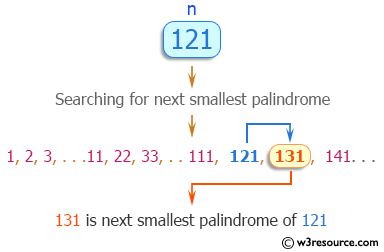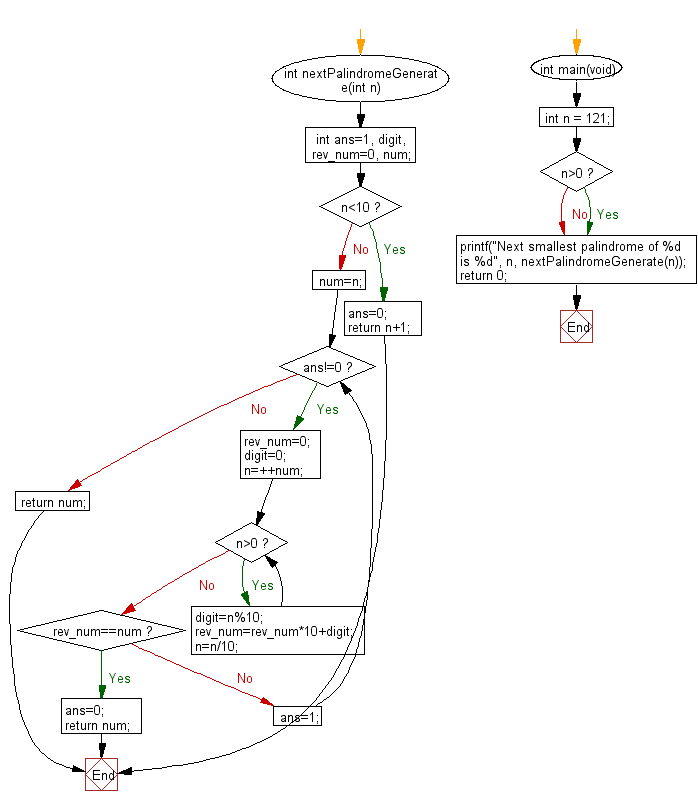﻿ C Program: Find next smallest palindrome of a given number - w3resource

# C Exercises: Find next smallest palindrome of a given number

## C Programming Mathematics: Exercise-23 with Solution

Write a C program to find next smallest palindrome of a given number.

From Wikipedia,
A palindrome is a word, number, phrase, or other sequence of characters which reads the same backward as forward, such as madam, racecar. There are also numeric palindromes, including date/time stamps using short digits 11/11/11 11:11 and long digits 02/02/2020. Sentence-length palindromes may be written when allowances are made for adjustments to capital letters, punctuation, and word dividers, such as "A man, a plan, a canal, Panama!".

Example:
Input: n = 121
Output: Next smallest palindrome of 121 is 131

Pictorial Presentation:Sample Solution:

C Code:

``````#include <stdio.h>
#include <stdlib.h>

int nextPalindromeGenerate(int n)
{
int ans=1, digit, rev_num=0, num;
if(n<10)
{
ans=0;
return n+1;
}
num=n;
while(ans!=0)
{   rev_num=0;digit=0;
n=++num;

while(n>0)
{
digit=n%10;
rev_num=rev_num*10+digit;
n=n/10;
}
if(rev_num==num)
{
ans=0;
return num;
}
else ans=1;
}
return num;
}
int main(void)
{
int n = 121;
if (n>0);
{
printf("Next smallest palindrome of %d is %d", n, nextPalindromeGenerate(n));
}
return 0;
}
```
```

Sample Output:

```Next smallest palindrome of 121 is 131
```

Flowchart:C Programming Code Editor:

Improve this sample solution and post your code through Disqus.

What is the difficulty level of this exercise?

Test your Programming skills with w3resource's quiz.

﻿